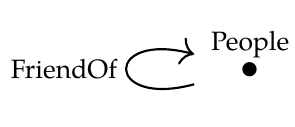OK, let's take \$$\mathcal{C}\$$ to be the people/friend-of schema from [Lecture 42](https://forum.azimuthproject.org/discussion/2236/lecture-42-chapter-3-transforming-databases).And \$$\mathcal{D}\$$ is the same category but without the friend-of arrow. There's only one choice for \$$G : \mathcal{D} \rightarrow \mathcal{C}\$$, which is the obvious inclusion functor.

Now consider a very simple database \$$H : \mathcal{D} \rightarrow \textbf{Set}\$$ that sends the object of \$$\mathcal{D}\$$ to the set \$$\\{\textrm{Alice}\\}\$$. What is its left Kan extension \$$\textrm{Lan}_G (H) : \mathcal{D} \rightarrow \textbf{Set}\$$?

It has to send the object of \$$\mathcal{C}\$$ to a set \$$P\$$ of people, but also send the friend-of arrow in \$$\mathcal{C}\$$ to an endomap on \$$P\$$ – and do this in a "free" way.

That means we need \$$\textrm{Alice} \in P\$$, but then we need to invent a friend for her, say \$$\textrm{Friend}_0 \in P\$$, and a friend for him \$$\textrm{Friend}_1 \in P\$$, and a friend for her \$$\textrm{Friend}_2 \in P\$$...

So \$$P\$$ is a countably infinite set (isomorphic to the natural numbers). There's a natural inclusion map \$$\\{ \textrm{Alice} \\} \rightarrow P\$$ but these sets certainly aren't iso.

Hence \$$\textrm{Lan}_G (H) \circ G\$$ is *not* iso to \$$H\$$.

I'm guessing that the issue here is that the inclusion functor \$$G\$$ is not full.